WEBINAR
Magnetic Levitation for Energy Harvesting
Thursday, June 22, 2023
Time
SESSION 1
SESSION 2
CEST (GMT +2)
03:00 PM
08:00 PM
EDT (GMT -4)
09:00 AM
02:00 PM
HOME / Applications / Magnetic Field along the Axis of a Cylindrical Coil

# Magnetic Field along the Axis of a Cylindrical Coil

Used Tools:## Physics

A cylindrical coil is used to create a strong magnetic field inside a domain. By wrapping the same wire many times around a cylinder, the magnetic field due to the current can become quite strong. The number of turnsrefers to the number of loops the cylindrical coil has. More loops will bring about a stronger magnetic field.

We will determine the magnetic fieldat a pointat the axis of a cylindrical coil of length, radius, turns, carrying current. According to the Biot-Savart law, the magnetic field of a current loop is:Whereis the vacuum permeability.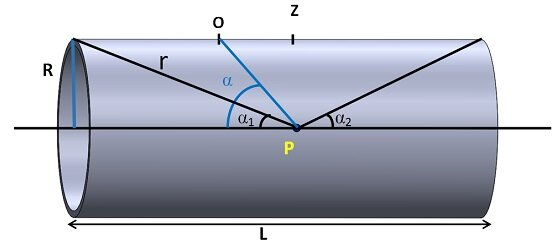Figure 1 - Schematic of a cylindrical coil

The net magnetic field on the axis of the cylindrical coil is the sum of the magnetic fields of all the loops.Divide the length of the cylinder into small elements of lengthto get the total field.The number of coil turns in a lengthis:Therefore:The total current in a lengthis:The magnetic field contributionat pointdue to each elementcarrying currentis:For each element of lengthalong the length of the cylinder, the distanceand the anglechange, while the value ofremains constant.From figure 1 we have:andExpression (2) can be differentiated as:Which results with:Formula forand formula forcan be substituted in the equation (1):Total magnetic fieldat any point on the axis can be obtained by integrating fromto:Hence this expression gives the magnetic field at point on the axis of a cylindrical coil of finite length.

## Model

A cylindrical coil of length 200mm, radius 5.5 mm , 100 turns, carrying current 10 A is modeled with Solidworks and simulated with Magnetostatic study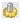in EMS.  Copper is assigned as a material to the cylinder body, while air covers  the inner air of the cylinder and the rest of the assembly. To get accurate magnetic field results, it is necessary to create sufficiently large air domain. To see how to assign material in EMS, see the “Computing capacitance of a multi-material capacitor” example.To learn how to define the air domain in EMS, consult the “Electric field inside the cavity of a charged sphere” example.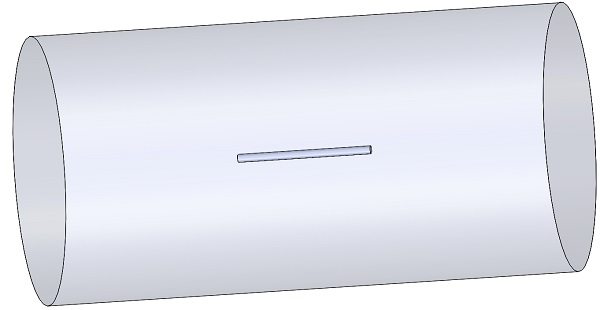Figure 2 - Solidworks model of the studied example

### Coil

To account for the magnetic field on the axis of the cylindrical coil, the cylinder should be used to define Wound Coil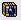with 100 turns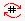and RMS current magnitude per turn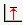of 10A.To prescribe the EMS Coil feature to the cylinder, it is necessary to have access to its cross-section surface. Therefore, the cylinder part should be split in two bodies. To learn how to do so, see “Magnetic field on axis of a current loop“example.Figure 3 shows the Entry and Exits ports of the Coil. In this case, Exit port is same as entry port.To show how to define the Wound Coil, see “Force in a magnetic circuit” example.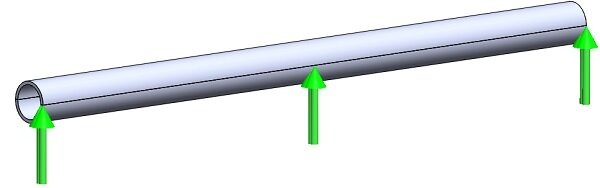Figure 3 - Entry and Exits ports of the Coil

### Mesh

Meshing is a very crucial step in the EMS simulation. Quality of the mesh in the inner air region and in the cylinder is of critical importance for accurate magnetic field calculation. To achieve a good accuracy without increasing the total number of mesh elements, a mesh control of 0.75 mm element size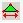, should be applied to the inner air and to the cylinder. To learn how to do so, see “Force in a magnetic circuit” example.

## Results

To display the variation of the magnetic field along the axis of the cylinder, before running the simulation:

1. In the Assembly, Select the ZX plane and sketch a line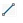along the z axis (axis of the coil), with a length equal to the length of the coil.
2. Then Insert/Reference geometry/Point and add a Reference point for each end of the line.
3. In the EMS feature tree, right click study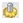and select Update geometry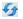.
4. Mesh and run the study.

Once the simulation is complete:

1. In the EMS feature tree, Under Results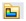, right click on the Magnetic Flux Density folder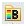and select 2D Plot then choose Linear.
2. The 2D Magnetic Flux Density Property Manager Page appears.
3.  In the Select points tab, click Import.
4. Click OK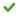.

The theoretical and EMS result of the magnetic flux density along the axis of the cylindrical coil are plotted in Figure 4.
It’s obvious that EMS result comply with the Biot-Savart law.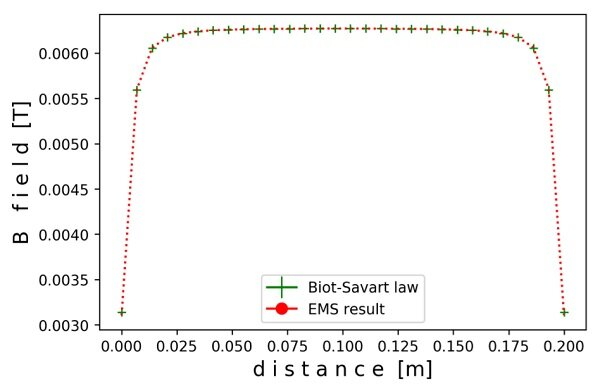Figure 4 - Comparison of EMS and theoretical results for magnetic flux density along the axis of a cylindrical coil